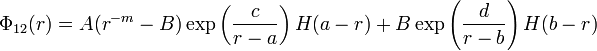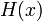# Dzugutov potential

The Dzugutov potential  is given by (Eq. 1):$\Phi_{12}(r) = A ( r^{-m} -B ) \exp \left( \frac{c}{r-a} \right) H(a-r) + B \exp \left( \frac{d}{r-b} \right) H(b-r)$

where$H(x)$ is the Heaviside step distribution.

## Parameters

The following parameters are taken from Table I

 m A c a B d b 16 5.82 1.1 1.87 1.28 0.27 1.94